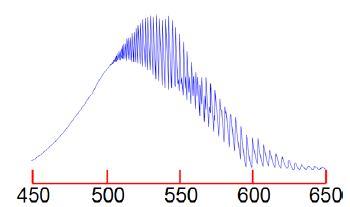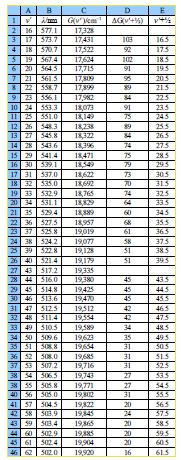Chemistry

# What is the visible Spectrum of Lodine?

The I2 molecule absorb at the red-end of the visible spectrum of lodine and thus appears bluish-purple. Figure shows the visible absorption spectrum of lodine taken using the teaching lab UV-visible spectrophotometer in Heriot-Watt University Chemistry Department.The fine structure is called a vibrational progression and it runs from at least 650 nm down to about 500 nm when it becomes continuous i.e. non-quantized. The vibrational level v’max. around 500 nm at which it becomes non-quantized is at the dissociation limit of the excited electronic state and thus the absorption spectrum changes from a quantized spectrum to a continuous spectrum with increasing energy of the photons absorbed. This allows us to measure in the lab the energy to jump from v = 0 of the ground electronic state to the Vmax the dissociation limit as shown in below Figure. Spectroscopy will be covered fully in your Chemistry lectures later in your degree.In the below figure the spreadsheet of the absorption spectrum of lodine has column B with the wavelengths ỳ/nm of the peaks for the transitions from v = 0 to the various V (column A) in the excited state. Wavelength is not proportional to energy but wavenumber G(V)/cm^-‘ is proportional to energy, and so the peaks of the vibrational progression are given in wavenumbers in column C of below figure.Type up your own spreadsheet for Columns A to E. The spectrum in below Figure shows that we only have peaks for the middle part of the progression, we don’t know the wavelength of the v = 0 to V = 0 transition as it is beyond the range of our spectrometer and so we need to look at the energy differences between neighbouring peaks. Column D of your spreadsheet should calculate ∆G(v’+1/2)

∆G (V+1/2) = G(V+1) – G (V)

You will notice that there is missing data for transitions to V = 41 and 42. Such missing data is common in science, so think carefully about how your spreadsheet can best handle the missing data points for the next step. The summation of these ∆G(V+ V2) is equal to the dissociation energy in the excited electronic state,  D’.

D’ = Ԑ ∆G (V+1/2)

The best way of proceeding when you don’t have the data from outside the spectrometer’s range is to fit a straight line through the graph of ∆G(v’i-1/2) against (v+1/2). From the equation for the line solve it to find the where the line crosses the x-axes i.e. the x-intercept. This extrapolation of a vibrational progression in spectroscopy is called the Birge-Sponer extrapolation. Extrapolation is always a worrying thing so finding the standard deviations of the gradient and intercept is very important. Once we have the x-intercept from the equation as well as the gradient and the y-intercept we can evaluate the Do’ graphically as the area under the triangle of the ∆G(v’+1/2) against (vi-1/2) line.